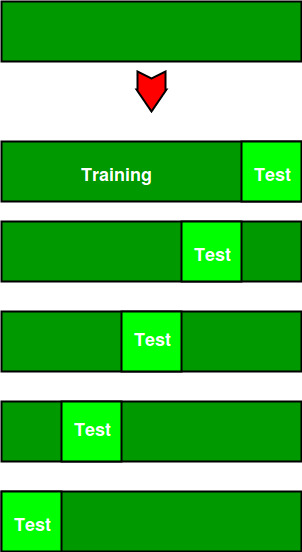# Cross Validation in Machine Learning

• Difficulty Level : Hard
• Last Updated : 07 Jan, 2020

In machine learning, we couldn’t fit the model on the training data and can’t say that the model will work accurately for the real data. For this, we must assure that our model got the correct patterns from the data, and it is not getting up too much noise. For this purpose, we use the cross-validation technique.

Cross-Validation

Cross-validation is a technique in which we train our model using the subset of the data-set and then evaluate using the complementary subset of the data-set.

The three steps involved in cross-validation are as follows :

1. Reserve some portion of sample data-set.
2. Using the rest data-set train the model.
3. Test the model using the reserve portion of the data-set.

Methods of Cross Validation

Validation
In this method, we perform training on the 50% of the given data-set and rest 50% is used for the testing purpose. The major drawback of this method is that we perform training on the 50% of the dataset, it may possible that the remaining 50% of the data contains some important information which we are leaving while training our model i.e higher bias.

LOOCV (Leave One Out Cross Validation)
In this method, we perform training on the whole data-set but leaves only one data-point of the available data-set and then iterates for each data-point. It has some advantages as well as disadvantages also.
An advantage of using this method is that we make use of all data points and hence it is low bias.
The major drawback of this method is that it leads to higher variation in the testing model as we are testing against one data point. If the data point is an outlier it can lead to higher variation. Another drawback is it takes a lot of execution time as it iterates over ‘the number of data points’ times.

K-Fold Cross Validation
In this method, we split the data-set into k number of subsets(known as folds) then we perform training on the all the subsets but leave one(k-1) subset for the evaluation of the trained model. In this method, we iterate k times with a different subset reserved for testing purpose each time.

```Note:
It is always suggested that the value of k should be 10 as the lower value
of k is takes towards validation and higher value of k leads to LOOCV method.
```

Example
The diagram below shows an example of the training subsets and evaluation subsets generated in k-fold cross-validation. Here, we have total 25 instances. In first iteration we use the first 20 percent of data for evaluation, and the remaining 80 percent for training([1-5] testing and [5-25] training) while in the second iteration we use the second subset of 20 percent for evaluation, and the remaining three subsets of the data for training([5-10] testing and [1-5 and 10-25] training), and so on.```Total instances: 25
Value of k     : 5

No. Iteration              Training set observations                     Testing set observations
1      [ 5  6  7  8  9 10 11 12 13 14 15 16 17 18 19 20 21 22 23 24]   [0 1 2 3 4]
2      [ 0  1  2  3  4 10 11 12 13 14 15 16 17 18 19 20 21 22 23 24]   [5 6 7 8 9]
3      [ 0  1  2  3  4  5  6  7  8  9 15 16 17 18 19 20 21 22 23 24]   [10 11 12 13 14]
4      [ 0  1  2  3  4  5  6  7  8  9 10 11 12 13 14 20 21 22 23 24]   [15 16 17 18 19]
5      [ 0  1  2  3  4  5  6  7  8  9 10 11 12 13 14 15 16 17 18 19]   [20 21 22 23 24]
```

Comparison of train/test split to cross-validation

Advantages of train/test split:

1. This runs K times faster than Leave One Out cross-validation because K-fold cross-validation repeats the train/test split K-times.
2. Simpler to examine the detailed results of the testing process.

 `# This code may not be run on GFG IDE ``# as required packages are not found. `` ` `# importing cross-validation from sklearn package.``from` `sklearn ``import` `cross_validation`` ` `# value of K is 10.``data ``=` `cross_validation.KFold(``len``(train_set), n_folds``=``10``, indices``=``False``)`